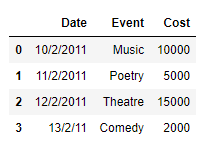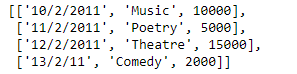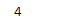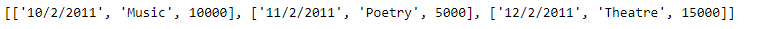# Create a list from rows in Pandas dataframe

Python list is easy to work with and also list has a lot of in-built functions to do a whole lot of operations on lists. Pandas dataframe’s columns consist of series but unlike the columns, Pandas dataframe rows are not having any similar association. In this post, we are going to discuss several ways in which we can extract the whole row of the dataframe at a time.

Solution #1: In order to iterate over the rows of the Pandas dataframe we can use `DataFrame.iterrows()` function and then we can append the data of each row to the end of the list.

 `# importing pandas as pd ` `import` `pandas as pd ` ` `  `# Create the dataframe ` `df ``=` `pd.DataFrame({``'Date'``:[``'10/2/2011'``, ``'11/2/2011'``, ``'12/2/2011'``, ``'13/2/11'``], ` `                    ``'Event'``:[``'Music'``, ``'Poetry'``, ``'Theatre'``, ``'Comedy'``], ` `                    ``'Cost'``:[``10000``, ``5000``, ``15000``, ``2000``]}) ` ` `  `# Print the dataframe ` `print``(df) `

Output :Now we will use the `DataFrame.iterrows()` function to iterate over each of the row of the given Dataframe and construct a list out of the data of each row.

 `# Create an empty list ` `Row_list ``=``[] ` ` `  `# Iterate over each row ` `for` `index, rows ``in` `df.iterrows(): ` `    ``# Create list for the current row ` `    ``my_list ``=``[rows.Date, rows.Event, rows.Cost] ` `     `  `    ``# append the list to the final list ` `    ``Row_list.append(my_list) ` ` `  `# Print the list ` `print``(Row_list) `

Output :As we can see in the output, we have successfully extracted each row of the given dataframe into a list. Just like any other Python’s list we can perform any list operation on the extracted list.

 `# Find the length of the newly  ` `# created list ` `print``(``len``(Row_list)) ` ` `  `# Print the first 3 elements ` `print``(Row_list[:``3``]) `

Output :Solution #2: In order to iterate over the rows of the Pandas dataframe we can use `DataFrame.itertuples()` function and then we can append the data of each row to the end of the list.

 `# importing pandas as pd ` `import` `pandas as pd ` ` `  `# Create the dataframe ` `df ``=` `pd.DataFrame({``'Date'``:[``'10/2/2011'``, ``'11/2/2011'``, ``'12/2/2011'``, ``'13/2/11'``], ` `                    ``'Event'``:[``'Music'``, ``'Poetry'``, ``'Theatre'``, ``'Comedy'``], ` `                    ``'Cost'``:[``10000``, ``5000``, ``15000``, ``2000``]}) ` ` `  `# Print the dataframe ` `print``(df) `

Output :Now we will use the `DataFrame.itertuples()` function to iterate over each of the row of the given Dataframe and construct a list out of the data of each row.

 `# Create an empty list ` `Row_list ``=``[] ` ` `  `# Iterate over each row ` `for` `rows ``in` `df.itertuples(): ` `    ``# Create list for the current row ` `    ``my_list ``=``[rows.Date, rows.Event, rows.Cost] ` `     `  `    ``# append the list to the final list ` `    ``Row_list.append(my_list) ` ` `  `# Print the list ` `print``(Row_list) `

Output :As we can see in the output, we have successfully extracted each row of the given dataframe into a list. Just like any other Python’s list we can perform any list operation on the extracted list.

 `# Find the length of the newly  ` `# created list ` `print``(``len``(Row_list)) ` ` `  `# Print the first 3 elements ` `print``(Row_list[:``3``]) `

Output :Whether you're preparing for your first job interview or aiming to upskill in this ever-evolving tech landscape, GeeksforGeeks Courses are your key to success. We provide top-quality content at affordable prices, all geared towards accelerating your growth in a time-bound manner. Join the millions we've already empowered, and we're here to do the same for you. Don't miss out - check it out now!

Previous
Next